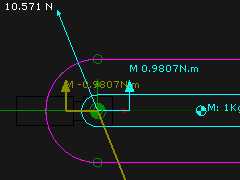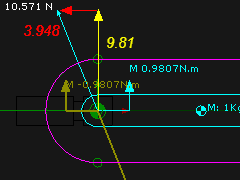﻿ Getting Started Tutorials - MechDesigner > Tutorial 13: Forces: Introduction  > Step 13.3: Rotating Rocker

# Step 13.3: A Rocker

### A Rotating Rocker – a Crank

 We connect a Linear-Motion FB to rotate the Rocker with a constant angular-velocity. This Step helps to understand: 1.Centripetal Acceleration and Force from the circular motion of the Center-of-Mass 2.Superposition of Gravitational Force and Centripetal Force 3.Why the Moment of the Centripetal-Force equals zero. STEP 1: Delete the Spring FB or Open the Spring FB dialog-box and make the Spring-Rate = 0 N/mm STEP 2: Add a Linear-Motion FB STEP 3: Connect a wire from the output-connector of the Linear-Motion FB to the input-connector of the Motion-Dimension FB. The Machine Speed Setting is 60RPM, or 1 Cycle/second = 2π radians/second. The mechanism below shows the forces that act at the Pin-Joint - the rotation axis of the Rocker.Addition of Vertical Forces acting on the Rocker (Point 2) : (↑+ve). ∑FV=0 : R2V(N) - 1(kg)*9.807(m/s/s) = 0;  R2V = 9.807N (upwards) Addition of Horizontal Forces acting on the Rocker (Point 2) : (→ +ve). ∑FV=0 : +R2H(N) + 1(kg)*0.1(m)*(2π)2(1/s/s) = 0;  R2H = -3.948N (to the left) Moments are: •An Anti-clockwise Moment that acts on the Rocker is 0.98Nm - as before. •The Centripetal Force acts through the center of rotation. It does not apply a moment to the Arm. Hence, the Moment is the same. The image to the left is the same as the image above. I have added to the image the Horizontal and Vertical Force Vectors that ACT-ON the Rocker The two components are: 1.Reaction to the Gravitational Force - it acts on the Rocker. Equal to 9.81N  2.Centripetal Force acts on the Rocker to the left. Equal to 3.948N The forces are perpendicular(⊥) when the Rocker is horizontal. Hence, we can use Pythagoras, to give 10.571N Ftotal = √(Fg2 + Fc2) (Total Force = SQRT(SQR(Gravitation Force) + SQR(Centripetal Force)) = SQRT((m.g)2 + (m.r.ω2)2) Total Force = √((1*g)2 + (1*0.1*(2π)2)2) = √(9.812 + 3.9482) = 10.571N